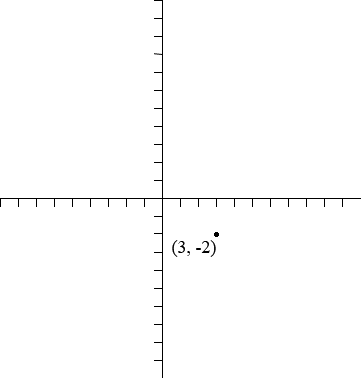SEARCH HOMEMath Central Quandaries & QueriesQuestion from Jude: M(3,-2) is the image of the point M under a translation by the vector (-5,2). Find the coordinates of MHi Jude,

The vector $(-5, 2)$ acts on the point $M$ in the plane by moving is 5 units to the left and 2 units upwards. The point $M$ is moved to $(3, -2).$Where was $M$ before the vector acted on it?